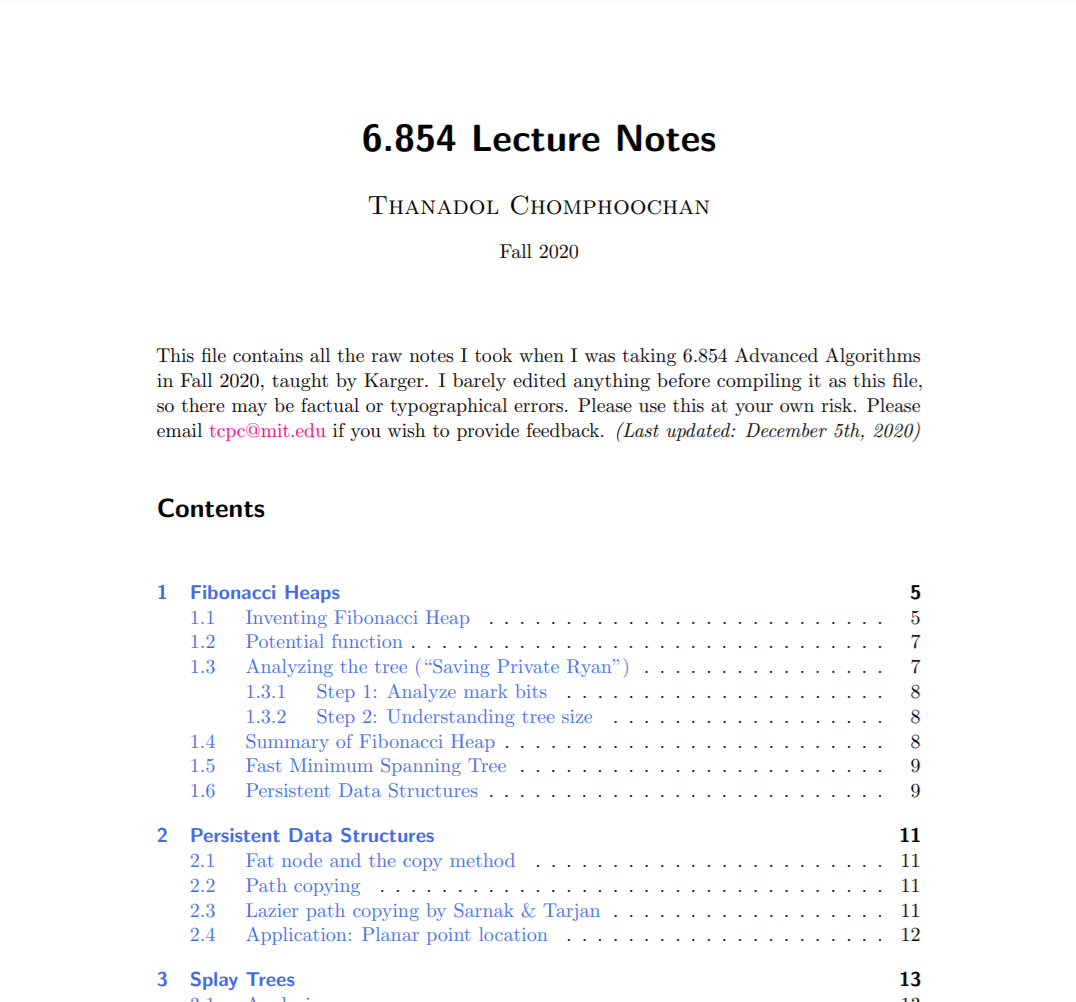# 6.854 Fall 2020 Lecture Notes

Saturday, December 5, 2020, 09:00 PMThe lectures are given according to this calendar from the official course website.

# Date Topic
1 Wed, Sep. 2 Course introduction. Fibonacci heaps. MST.
2 Fri, Sep. 4 Fibonacci heaps. MST. Persistent Data Structures Intro.
3 Wed, Sep. 9 Persistent Data Structures. Splay trees intro.
4 Fri, Sep. 11 Splay trees.
5 Mon, Sep. 14 Dial’s Algorithm. Tries. Multi-level buckets.
6 Wed, Sep. 16 van Emde Boas Queues and Hashing
7 Fri, Sep. 18 Universal Hashing. Perfect Hashing. Begin Network Flows.
8 Mon, Sep. 21 Network Flows: Charaterization. Augmenting Paths. Max Flow Min Cut Theorem.
9 Wed, Sep. 23 Network Flows: Maximum augmenting path. Capacity Scaling.
10 Fri, Sep. 25 Network Flows: Strongly polynomial algorithms. Blocking Flows.
11 Mon, Sep. 28 Min-Cost Flows: Feasible prices.
12 Wed, Sep. 30 Min-Cost Flows
13 Fri, Oct. 2 Finish Min-Cost Flows and Introduction to Linear Programming.
14 Mon, Oct. 5 Linear Programming: Structure of Optima.
15 Wed, Oct. 7 Linear Programming: Strong Duality.
16 Fri, Oct. 9 Linear Programming: Strong Duality and Duality Applications.
17 Tue, Oct. 13 Linear Programming: Duality Applications.
18 Wed, Oct. 14 Linear Programming: Simplex Method.
19 Fri, Oct. 16 Linear Programming: Ellipsoid Method.
20 Mon, Oct. 19 Introduction to Approximation Algorithms.
21 Wed, Oct. 21 Approximation Algorithms: Greedy approaches. Enumeration and FPAS (scheduling)
22 Fri, Oct. 23 Approximation Algorithms: Enumeration, Rounding, Metric TSP.
23 Mon, Oct. 26 Approximation Algorithms: Relaxations
24 Wed, Oct. 28 Approximation Algorithms: Randomized Rounding
25 Fri, Oct. 30 Computational Geometry I.
26 Mon, Nov. 2 Computational Geometry II: Voronoi Diagrams
27 Wed, Nov. 4 Online Algorithms: Ski Rental, Paging.
28 Fri, Nov. 6 Online Algorithms: Paging, Adversaries, Randomization
29 Mon, Nov. 9 Online Algorithms: Adversaries, Randomization, k-server.
30 Fri, Nov. 13 The k-server problem and External Memory Algorithms.
31 Mon, Nov. 16 External Memory Algorithms.
32 Wed, Nov. 18 Parallel Algorithms.
33 Fri, Nov. 20 Parallel Algorithms.